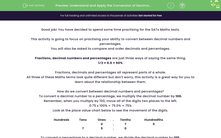# Understand and Apply the Conversion of Decimal Numbers to Percentages 1

In this worksheet, students will practice a key skill for the reasoning SATs Maths test papers, the ability to convert between decimal numbers and percentages. They will also be challenged to order and compare decimals and percentages.This content is premium and exclusive to EdPlace subscribers.Key stage:  KS 2

Curriculum topic:   Exam-style Questions: SATs Maths

Curriculum subtopic:   Exam-Style Questions: Decimal Conversion

Difficulty level:#### Worksheet Overview

Good job! You have decided to spend some time practising for the SATs Maths tests.

This activity is going to focus on practising your ability to convert between decimal numbers and percentages.

You will also be asked to compare and order decimals and percentages.

Fractions, decimal numbers and percentages are just three ways of saying the same thing.

1⁄2 = 0.5 = 50%

Fractions, decimals and percentages all represent parts of a whole.

All three of these Maths terms look quite different but don't worry, this activity is a great way for you to learn about the relationship between them.

How do we convert between decimal numbers and percentages?

To convert a decimal number to a percentage, we multiply the decimal number by 100.

Remember, when you multiply by 100, move all of the digits two places to the left.

0.75 x 100% = 75.0% = 75%

Look at the place value chart below to see the movement of the digits.

 Hundreds Tens Ones . Tenths Hundredths 0 . 7 5 7 5 . 0

To convert a percentage to a decimal number, we divide the decimal number by 100.

Remember, when you divide by 100, move all of the digits two places to the right.

75% ÷ 100 = 0.75

Look at the place value chart below to see the movement of the digits.

 Hundreds Tens Ones . Tenths Hundredths 7 5 . 0 0 . 7 5

Over to you now to have a go at some practice questions...good luck!

### What is EdPlace?

We're your National Curriculum aligned online education content provider helping each child succeed in English, maths and science from year 1 to GCSE. With an EdPlace account you’ll be able to track and measure progress, helping each child achieve their best. We build confidence and attainment by personalising each child’s learning at a level that suits them.

Get started